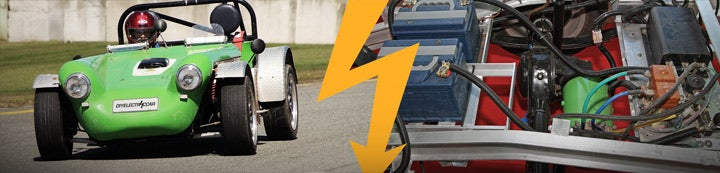# math

1. ### Heavy Mathematical magic - Calculating Acceleration from Torque

Technical Discussion
Okay, so I have a wheel of radius r (Meters), attached to a vehicle of weight M (Kilograms). If I apply a constant torque T (Newton-Meters), how much acceleration do I get? My shaky math comes out to: Inertia I=W*r² Acceleration = T/I (In Radians/sec) Therefore Acceleration = (T/I)*r (In...